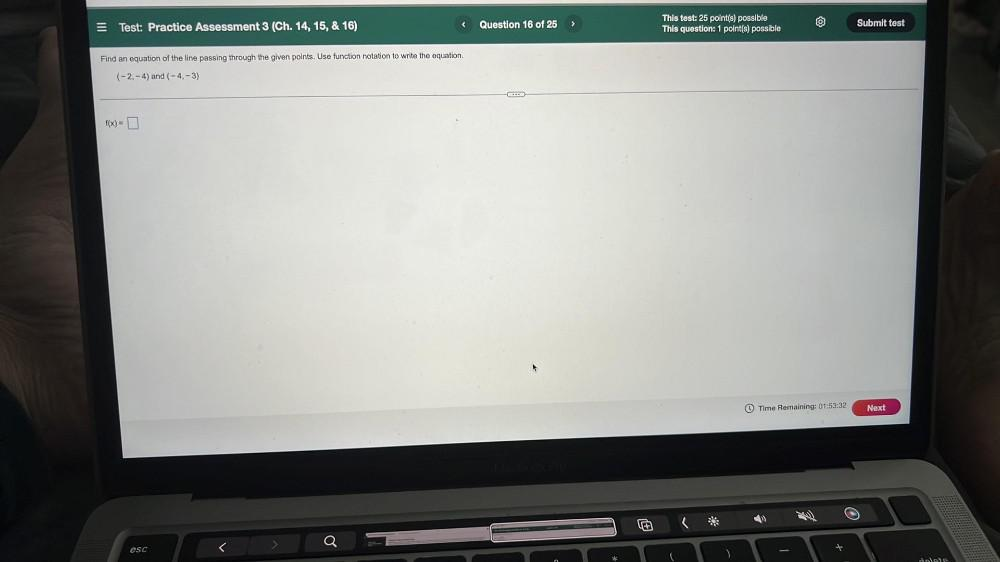Question:

# Find an equation of the line passing through the given points. Use function notation to write the equation. (-2,-4) and (-4,-3) f(x) = ___Find an equation of the line passing through the given points. Use function notation to write the equation. (-2,-4) and (-4,-3) f(x) = ___